# Physical Chemistry : Heat Transfer

## Example Questions

### Example Question #1 : Thermochemistry And Thermodynamics

A chemical reaction is run in whichof work is done by the system and the internal energy changes by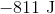. What is the total amount of heat transferred?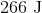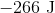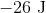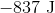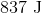Explanation:

The First Law of thermodynamics states that for a system that only exchanges energy by heat or work: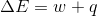Work is done by the system, therefore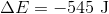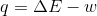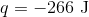### Example Question #1 : Thermochemistry And Thermodynamics

An automobile engine provides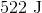of work to push the pistons and generates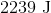of heat that must be carried away by the cooling system. What is the change in the internal energy of the engine?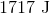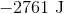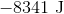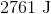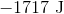Explanation:

The First Law of thermodynamics states that for a system that only exchanges energy by heat or work:The heat is given off by the system, so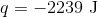. Similarly, work is being done by the system, so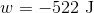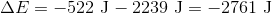### Example Question #1 : Thermochemistry And Thermodynamics

A chemical reaction is run in which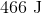of heat is absorbed and the internal energy changes by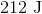. What is the amount of work done?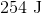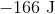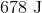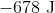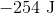Explanation:

The First Law of thermodynamics states that for a system that only exchanges energy by heat or work:Heat is absorbed by the system, therefore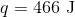So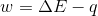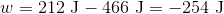done by the system.

### All Physical Chemistry Resources